﻿ Industrial Robot Backlash Fault Diagnosis Based on Discrete Wavelet Transform and Artificial Neural Network

### Industrial Robot Backlash Fault Diagnosis Based on Discrete Wavelet Transform and Artificial Neural ...

American Journal of Mechanical Engineering

## Industrial Robot Backlash Fault Diagnosis Based on Discrete Wavelet Transform and Artificial Neural Network

Alaa Abdulhady Jaber1, 2,, Robert Bicker1

1Mechanical and Systems Engineering, Newcastle University, Newcastle upon Tyne, UK

2Mechanical Engineering Department, University of Technology, Baghdad, Iraq

### Abstract

Industrial robots are commonplace in production systems and have long been used in order to improve productivity, quality and safety in automated manufacturing processes. An unforeseen robot stoppage due to different reasons has the potential to cause an interruption in the entire production line, resulting in economic and production losses. The ability to continuously monitor the status and condition of robots has become a research issue in recent years and is now receiving considerable attention. Thus, the main aim of this research is to develop an intelligent condition monitoring system to diagnose the most common faults (backlash) that could be progressed in the gearbox of industrial robot joints. For accurate fault diagnosis, time-frequency signal analysis based on the discrete wavelet transform (DWT) is adopted to extract the most salient features related to faults, and the artificial neural network (ANN) is used for faults classification. A data acquisition system based on National Instruments (NI) software and hardware was developed for robot vibration analysis and feature extraction. An experimental investigation was accomplished using the PUMA 560 robot. Firstly, vibration signals are captured from the robot when it is moving one joint cyclically. Then, by utilising the wavelet transform, signals are decomposed into multi-band frequency levels starting from higher to lower frequencies. For each of these levels the standard deviation feature is computed and used to design, train and test the proposed neural network. The developed system has showed high reliability in diagnosing several seeded backlash levels in the robot.

• Alaa Abdulhady Jaber, Robert Bicker. Industrial Robot Backlash Fault Diagnosis Based on Discrete Wavelet Transform and Artificial Neural Network. American Journal of Mechanical Engineering. Vol. 4, No. 1, 2016, pp 21-31. http://pubs.sciepub.com/ajme/4/1/4
• Jaber, Alaa Abdulhady, and Robert Bicker. "Industrial Robot Backlash Fault Diagnosis Based on Discrete Wavelet Transform and Artificial Neural Network." American Journal of Mechanical Engineering 4.1 (2016): 21-31.
• Jaber, A. A. , & Bicker, R. (2016). Industrial Robot Backlash Fault Diagnosis Based on Discrete Wavelet Transform and Artificial Neural Network. American Journal of Mechanical Engineering, 4(1), 21-31.
• Jaber, Alaa Abdulhady, and Robert Bicker. "Industrial Robot Backlash Fault Diagnosis Based on Discrete Wavelet Transform and Artificial Neural Network." American Journal of Mechanical Engineering 4, no. 1 (2016): 21-31.

 Import into BibTeX Import into EndNote Import into RefMan Import into RefWorks

12345
Prev Next

### 1. Introduction

The industrial robot is defined as a reprogrammable multifunctional manipulator designed to move material, parts, tools, or specialized devices through variable programmed motions for the performance of a variety of tasks . The mechanical structure of a standard industrial robot is composed of number of links and joints. Links are the main bodies that make the mechanism and are connected to each other by joints. However, condition monitoring (CM) is defined as a type of maintenance inspection where an operational asset is monitored and the data obtained is then analysed to detect signs of degradation, diagnose the causes of faults, and also predict how long it can be safely or economically run , and it is used for health monitoring of different machines. Industrial robots are extremely complex mechanism and hence the application of CM for them differs from that of ‘simple’ rotating machinery. This is basically due to the instantaneous change of geometrical configuration of the robot arm. There are two approaches to condition monitoring, which are model-based (based on the mathematical model of the system) and model-free (depends on the features extracted from the measured system data for building a model that represents the system). Either of these approaches or a combination of both has been adopted in industrial robot condition monitoring. For example, Filaretov, et al.  used a nonlinear model to address problems of fault detection and isolation in robot manipulators. Algebraic functions were implemented to design the nonlinear diagnostic observer, which was able to dispense with the linearization in nonlinear models to avoid model errors. The robot modeling was conducted using Matlab in discrete time. In another paper, a model-based fault detection and isolation (FDI) scheme for rigid manipulators was designed which depends on a suboptimal second-order sliding-mode (SOSM) algorithm . In order to make the procedure of FDI possible, an input signal estimator and output observers were adopted and SOSM was used to design the input laws for the observers. Experimental work and theoretical simulations were accomplished with a COMAU SMART3-S2 robot manipulator, and the results showed that the scheme has a good ability to detect and identify faults. On the other hand, the proposed scheme was not able to deal with multiple faults in more than one actuator or sensor, and is also neglected elastic effects in the robot.

However, because precise mathematical models for complex systems like a robot are difficult to obtain, model-free methods based on artificial intelligence (AI) or statistical approaches have become prevalent choices for robot health monitoring. For instance, a technique using only one accelerometer mounted at the robot tip has been applied for the online fault diagnosis in the 4 degree of freedom (DOF) SCARA robot . The tip acceleration was calculated using a dynamic model of the robot, and was used as a reference. By comparing the experimental tip acceleration with the reference, the condition of the robot could be identified. In contrast, another study used more than one sensor for robot joint condition monitoring . The objectives were to extract the vital features directly from the measured acceleration signals, and to try to specify defects by finding properties dependent on fault size. Signals were analyzed from the robot joints without error, and subsequently from joints having backlash, using nonlinear dynamics and statistical tools. The proposed system was validated using three robot types: spherical robot arm; SCARA robot arm; revolute robot arm, and on different joints. The authors used the pattern recognition principle with nonlinear autoregressive (NAR) analysis for the detection of defect from the data, and acceptable performance was demonstrated.

In this research, however, a condition monitoring system is developed, based on vibration signal analysis, to detect and diagnosis the most common fault (backlash) that could be progressed in the robot joints. The developed system is based on the combination of the discrete wavelet transform (DWT) for feature extraction, as it is suitable for nonstationary and transitionary signal analysis, and the artificial neural network (ANN) for feature classification. Principally, this work is a continuation to a previously conducted researches by the authors for industrial robot health monitoring [7, 8, 9].

### 2. Discrete Wavelet Transform (DWT)

After a signal is captured, a large number of signal processing techniques can be utilized to extract the most sensitive and interesting features concerning defects. Signal processing techniques are classified as time domain, frequency domain, and time-frequency domain methods. In fact, choosing the most suitable method for each specific task represents a major challenge in fault diagnosis. However, industrial robots are required to function under a wide range of joint speeds and variable loading within a large working area and varying joint articulation. Also, the typical cycle of robot motion starts with an acceleration from the initial position, then moving at constant speed, and finally deceleration towards the end position, which means movement at a time-varying speed [10, 11]. This motion makes the robot a highly non-linear dynamic system and introduces the non-stationary phenomenon in the captured vibration signal, and this will be more complicated if a fault is progressed in the robot. Using the conventional signal analysis techniques, such as the fast Fourier transform (FFT), to process such signals with transiently nature is not feasible for accurate robot fault diagnosis. Therefore, several methods of signal processing have been developed to cope with this category of signals, such as joint time-frequency techniques using the DWT or short time Fourier transform, because of their capabilities of signal representation in both the frequency and time domains [12, 13]. Also, time-frequency methods can give interesting information in regards to energy distribution over frequency bands.

However, DWT is superior to STFT and was introduced to overcome the resolution limitations of the STFT. The main difference between the DWT and the STFT is that the former has varying window lengths, and it represents the signal as a sum of wavelets at different scales . The concept of the DWT is that filters with different cut-off frequencies are utilized to analyze the signal at different scales. Firstly, the signal is passed through a high-pass filter to analyze high frequencies, and then it is passed through a low-pass filter to analyze low frequencies. Generally, by using the DWT, a multi-resolution analysis can be performed at different frequency bands with different resolutions by decomposing the time domain signal [12, 14]. Two sets of functions are employed in the DWT. These are called the wavelet function and the scaling function, which are associated with the high-pass (HP) and low-pass (LP) filters respectively. At the first level, the original signalis decomposed by passing it through both of these filters and emerges as two signals, each one having the same number of samples as the original signal, and are termed as coefficients. In order to keep the total number of coefficients in the produced filtered signals equal to the original signal samples they are then down-sampled by a factor of 2, by keeping only one sample out of two successive samples. Thus, the extracted signal coefficients from the HP filter and after down sampling are called the detail coefficients of the first level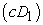. These coefficients contain the high frequency information of the original signal, whilst, the coefficients that are extracted from the LP filter and after the down sampling process are called the approximation coefficients of the first level. The low frequency information of the signal is hidden in these coefficients. This can be expressed mathematically as :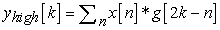(1)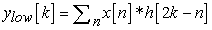(2)

where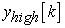andare the outputs of the high-pass and low-pass filters respectively, after down-sampling by 2. After obtaining the first level of decomposition, the above procedure can be repeated again to decomposeinto another approximation and detail coefficients. This procedure can be continued successively until a pre-defined certain level up to which the decomposition is required to be found. At each decomposition level, the corresponding detail and approximation coefficients have specific frequency bandwidths given byfor the approximation coefficientsand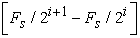for the detailed onewhereis the sampling frequency [12, 15]. However, at every level, the filtering and down-sampling will result in half the number of samples (half the time resolution) and half the frequency band (double the frequency resolution). Also, due to the consecutive down sampling by 2, the total number of samples in the analysed signal must be a power of 2 . By concatenating all coefficients starting from the last level of decomposition, the DWT of the original signal is then produced, and it will have the same number of samples as the original signal. A schematic diagram illustrates how the multi-level decomposition is performed shown in Figure 1. The number of decomposition levels is identified by the lowest frequency band needed to be traced, and a higher number of decomposition levels are required if very low frequency band is investigated. However, the highest decomposition level that can be achieved is up to that the individual details consist of a single sample .Download asVeiw figureFigures index
Figure 1. Signal decomposition using discrete wavelet transform

Once the approximation and detail coefficients are computed to different levels of decomposition, it becomes possible to reconstruct the approximation and detail signals at each level, in order to extract features, such as standard deviation, related to the frequency bands in each level. Each signal, however, will have the same number of samples as the original signal but with a definite frequency band. This can be achieved by up-sampling the approximation (or details) coefficients by two, since they were produced previously by down sampling by 2, and then passing them through high- and low-pass synthesis filters. In this work and based on a preliminary robot vibration analysis was established that eight decomposition levels are sufficient for analysing the robot vibration signals, as explained later; also, Daubechies and Symelet wavelet families are recognized as very effective in vibration signal analysis and have various wavelet orders, thus, in this project Daubechies’s second order (db2), which is the same as Symelet’s second order (sym2), has been selected.

### 3. Artificial Neural Network (ANN)

The ANN is computational structure inspired by the data processing and learning ability of biological neurons in the nervous system. It has a summation type of structure that consists of several layers of artificial neurons, which integrate the functionality of both memory and computation, designed to emulate the biological neurons. Each input signal flows through a gain or weight, called a synaptic weight or connection strength and can be positive or negative, integer or non-integer. Figure 2 illustrates a typical representation of an artificial neuron, with connection weights.Download asVeiw figureFigures index
Figure 2. Artificial neuron with connection weights

Whererepresent the input vector to the neuron with associated weights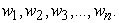The bias,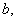(sometimes called threshold) is often connected to the neuron and it introduces an offset to the transfer function so that even if the input were zero the neurons would still have an output . Typically, the bias is set to a value of 1. However, the input to the neuron, which is called net, will be the summation of the multiplied inputs with their corresponding weights, and can be written as: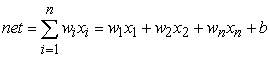(3)

where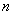is the number of inputs to the neuron. The resultant net is then passed through an activation function, sometimes called the transfer function that can be linear or nonlinear, to produce the output which can be represented as:(4)

Neural networks come in many forms and the structure of the connections between neurons and the computation it performs vary between the different neural models. ANNs are generally classified as feed-forward, e.g. the multilayer perceptron neural network (MLPN), and feed-back (or recurrent) types, such as Hopfield network. In the feed-forward class, the signals travel only in the direction from the input to the output, whereas in recurrent neural network (RNN), the signals can flow in the forward as well as backward or lateral direction. However, the main characteristic of a neural network is that, when the training process is performed well, the mapping formed by the network can show its capability for generalization beyond the training data and not to memorise the training data. The majority of fault diagnosis applications utilizing ANNs utilize a feed-forward architecture, with the MLPN being most popular and thus it adopted for use in this research.

### 4. The Experimental Set-up

The experimental work has been performed using the PUMA 560 robot, which is a PC-controlled serial manipulator with six revolute joints/degrees of freedom (DOF). It is an old (>25 years), but functional, multi-joint robot and was used in this work. Three single axis, analogue outputs, 70g, MEMS accelerometers type ADXL001 from Analog Device have been used for signal capture. These accelerometers have been put together in a configuration that allows the vibration signals in the X, Y, and Z directions to be measured using a specially designed adapter. It may be assumed that, to detect the abnormalities in a machine, the sensors should be located near expected damage locations. However, there are cases where the damage is more recognizable at other locations on the machine. So, prior to acquiring the data for fault detection, it is crucial to locate the sensitive positions on the robot. A preliminary analysis was performed and it was found that the best position to fix the accelerometer on the robot is near to the wrist joint, as shown in Figure 3. The signals from the accelerometers are fed to a 14-bit NI data acquisition card (DAQ) type USB-6009.Download asVeiw figureFigures index
Figure 3. The experimental set-up

In terms of data acquisition software, many functions have been provided by LabVIEW, such as the interface with Matlab and C language, which make graphical programming in LabVIEW more flexible. Furthermore, Matlab software has many specialized, efficient, and easy-to-learn toolboxes, and so some of commends in the wavelet analysis toolbox are used here. This toolbox offers many options in helping to accomplish wavelet analysis, such as specifying the number of decomposition levels and selecting a suitable wavelet family. Therefore, combining the capabilities of Matlab in data processing and the advantages of LabVIEW graphical programming, the virtual instrument produced will have more capabilities and greater flexibility. In order to achieve the LabVIEW and Matlab interface, LabVIEW offers a Matlab script node to call a programme written in the Matlab language, which was used in this work. A sample from the prepared LabVIEW code is shown in the Figure 4. The LabVIEW block diagram begins with a for loop. However, many parameters in the code have to be tuned properly for accurate wavelet analysis, such as which wavelet family, number of decomposition levels, and this was achieved using (wavedec) command. Researchers who want to accomplish feature extraction using wavelet are advised to read in detail the (wavelet toolbox user guide) .

In the experimental process and since the faults are simulated in joint 3, as discussed in the following section, the robot was programmed to oscillate over its full range of movement (270 degree), as some of the robot faults may exhibit direction-dependent symptoms . This is also in order to relate the produced vibration to the moving joint’s transmission components such as gears and bearings. Vibration signals from the three axes of measurements are captured at a sampling frequency of 1031 Hz with sample size equal to 4096. This frequency is about ten times higher than the 11th natural frequency of the robot, based on a conducted preliminary robot modal (frequency response function) analysis, which fulfils the sampling theory that requests the sampling frequency to be at least two times higher than the highest system frequency . Thus, eight wavelet decomposition levels are found to be sufficient for analysing the robot vibration signal. Statistical features are computed from each detail signal produced after the DWT analysis; these features are utilized for designing a neural network for fault diagnosis.Download asVeiw figureFigures index
Figure 4. Part from the prepared LabVIEW code

### 5. Robot’s Mechanical Construction and Faults

Joints in industrial robots are commonly actuated by electrical motors. Permanent magnet servomotors are a popular choice to produce the driving force to move robot joints because of their easy operation, high power density and performance . In general, servomotors are electromechanical components in which faults can originate for electrical, mechanical and other external reasons. In order to transform motor power to the robot joints, mechanical reduction gears in the transmission system are normally used. The power is then transmitted from the input to the output shaft through the gear contacts and mesh. However, this paper concentrates on fault detection in the elbow joint (joint 3) of the robot, which is shown in Figure 3 above. The mechanical construction of this joint is illustrated in Figure 5; it consists of a two-stage gear train system housed in the end of the upper arm and connected to a DC motor by a drive shaft. There is a bevel pinion on the input shaft meshed with a bevel wheel on one end of an idler shaft. Also, a spur pinion at the other end of the idler shaft engages with a spur wheel fixed to the forearm, and therefore rotates the forearm around the elbow axis. A number of deep groove ball bearings are used to carry the input and idler shafts.

The main fault mechanisms that may appear in the joint gearbox are basically the same as those arising in other types of gearboxes, such as backlash in the gearbox, and gear and bearing faults. The most common types of gear tooth failure are scuffing, cracking, macro- and micro-pitting, wear, bending fatigue, and fracture due to overload. Moreover, since gears are normally supported on rolling element bearings, faults in these bearings such as wear in the inner or outer races represent another typical type of fault in the robot’s transmission system. However, the focus of this paper is limited for diagnosing only the backlash faults, which is defined as “the clearance between the non-working flanks of the teeth of a gear pair when the working flanks are in contact” .Download asVeiw figureFigures index
Figure 5. Schematic diagram of joint 3 (elbow joint)
5.1. Backlash Faults Simulation

It is accepted that the best way to introduce a fault in the robot is by operating the robot to execute a specific task for a considerable amount of time until a fault is developed. This method, however, has not been followed in this work primarily because it may resulting other joints of the robot failing. The faults that will be introduced here are not truly representational of real faults in the robot, since in a practical situation the majority of robot faults are progressive. However, this methodology has important advantages such as different types of fault can be produced in different components of the joint 3 gearbox and the degree of fault severity can be controlled.

The input and idler shafts in joint 3 (Figure 5) are carried inside the robot structure using hollow and eccentric shafts. There are three slots on the surfaces of these hollow shafts, as shown in the Figure 6, utilized to adjust the backlash in the bevel and spur gears pair. Two of these slots, diametrically positioned, are used for changing the centre distance in the spur gear pair and for changing the mounting distance of bevel wheel gear, and are used to varying the backlash in each gear set. These changes can be achieved by using adapted grub-screws which fit into the slots. The circumfrentional slot in the two shafts is used to adjust these gears axially to control the contact pattern between meshed gears, and is accomplished using an eccentric grub-screw, shown in Figure 6. Three levels of backlash were introduced in the spur gears, starting from very high level (large clearance between the gears = 0.92 mm), then intermediate level (small clearance = 0.8 mm) and interference (0.56 mm), where in the healthy condition the backlash is 0.68 mm. It was found that a total of approximately five grub-screw turns are required to move from high backlash level to interference level. Also, depending on the shaft and grub-screw dimensions, simple calculations was done and established that every one screw turn is roughly equivalent to increasing or decreasing the backlash by 0.072 mm. This is an important finding, since it helps to get a preliminarily indication about the number of turns required to simulate different backlash values in this work. The interference case should not develop during the normal operation of the robots, but it could occur if the gear mesh are assembled/adjusted incorrectly after maintenance. The optimum backlash setting was first recorded before introducing any fault type on the robot, using a dial-gauge indicator, as shown in Figure 7, and each time the backlash value is calibrated with respect to this optimum value using the same measurement technique .Download asVeiw figureFigures index
Figure 6. Input and idler shafts and their backlash adjustment grub-screwsDownload asVeiw figureFigures index
Figure 7. Robot backlash measurement 

### 6. Robot Vibration Analysis

Selected results of the multi-resolution signal analysis using DWT extracted from the LabVIEW code are shown in Figures 8. This figure represent the analyzed signal from the X-axis accelerometer when the robot is healthy and when the highest backlash level is introduced in the robot.Download asVeiw figureFigures index
Figure 8. DWT analysis of the X-axis vibration signals from the robot arm when it is healthy and high backlash fault simulated (auto scaled)

There are 10 sub-figures for each measurement axis, as in Figure 8; the first sub-figure is the original signal before the DWT is applied, while the rest are the eight constructed sub-signals from the detail coefficients (D1, D2, D3, D4, D5, D6, D7, and D8), and one from the approximation coefficient (A8), where each one contains a specific frequency band presented in the upper left corner of each sub-figure. These bands were computed based on the formulas that were presented in Section 2. Generally, accelerometers provide mixed information about vibration and movement acceleration in the robot . The vibration signal is made up of high frequencies, whereas movement acceleration signals are associated with low frequencies . After conducting the DWT on the original signals and by investigating the produced sub-signals it was concluded that the approximation signals (A8) in the three axes are mostly related to the robot movement and not to its vibration components. Consequently, the approximation signals do not carry any useful information regarding robot faults and are not considered important in the present work. However, extracting the robot movement acceleration using DWT could be employed in future work to estimate the kinematics of the industrial robots .

Always vibration of multi-stage gearboxes, as in the robotic system, is very complex and composed of different frequencies, including gear mesh, bearings, and running-speed frequencies. The vibration severity is also connected with the excited resonance frequencies of the robot. The high speed of the robot gears causes cyclic excitation of these resonance, leading to some periodic fluctuations with amplitude proportional to the fault severity. This can clearly be seen in the analysed signals. The high amplitude components present in the signals were observed when the robot changes the rotating direction of the joints. This is leading to developing number of impact between the mating pairs, due to the contact force between teeth.

It was anticipated that by increasing the backlash the vibration level would increase in the robot, but the opposite has been found. This can clearly be seen by comparing the vibration signals produced with the high backlash level to the healthy robot condition. For instance, in the case of higher backlash the amplitudes of vibration signals are lower than those of the healthy case; this also corresponds with the results of research published by Bicker, et al. . The amplitudes of vibration increase when the backlash level is reduced, until the increase became more significant in the interference case. Although the presence of backlash in the transmission system can cause a transient impact at the reversal of motion, which will lead to an undesirable level of vibration, high backlash between gears will allow more lubricating grease to enter between the mating teeth leading to damping of the vibration. Whereas, the tight gear mesh leads to the lubricant being squeezed out of the mating teeth and the system heats up due to friction between the teeth, resulting in increased vibration.

The sub-signals from the DWT analysis could be directly used as inputs to the classifier, which will be the ANN. However, feeding huge amounts of data, represented by 4096 samples from each sub-signal, into the classifier will make the classification process more complex and computationally intensive. To overcome this, a post processing stage is needed to take out significant parameters from the sub-signals, called the feature extraction stage, and will significantly reduce the data set size required to be passed to the classifier for correct fault diagnosis. Based on this, several statistical functions, e.g. the root mean square (RMS), standard deviation (STD), and kurtosis, are applied to the detail signals from the three axes of measurement, since approximation signals are neglected, as discussed earlier. These features are extracted in real-time after the data is captured and DWT analysis is applied using the designed LabVIEW code. However, if all computed features are used for fault classification, this will also involve significant amount of data for the classifier. Thus, only the most sensitive feature was utilized. The STD feature, which is normally used as a measure of extent of variation of the processed data and has the same units as the data, was found to be the most faults sensitive; and hence it was selected for comparison among fault severities. Therefore, 8 STD features from each axis signal are obtained, and by joining all features from the three axis signals together the input vector to the classifier will be constituted from 24 features.

Figures 9 shows the computed SDTs from the eight detail signals from accelerometer axes X, Y, and Z when different backlash levels are simulated as well as the result extracted from the detail signals of the robot healthy condition. The high backlash case records the smallest STDs at all detail levels among the healthy and other backlash cases and this is similar in all the three axes. These values are increased by reducing the backlash until they become significantly high when the backlash is completely removed (interference case). This, as discussed previously, is attributed to vibration damping due to entering the lubricant grease between the mating teeth when excessive clearance between them and squeeze it when they are interfered. The STD vectors at healthy and other backlash levels are clearly separated in X-axis, and also clear separation is shown up to D5 in Z-axis, while they are almost squeezed together in Y-axis. However, the Y-axis results will not be ignored and will be included in the input vectors to the classifier, as sure the STDs in Y-axis will give a clear indication about a fault if it is developed, for example, in other joints or parts. Generally speaking, if the STD vectors are very well isolated, the problem of fault classification will be easier when ANN is used, the false diagnosis rate is reduced, and also the designed ANN will be of small size helping in lowering the required processing power.

### 7. Design of Multilayer Perceptron Neural Network (MLPN) for Fault Diagnosis

To model the nonlinear characteristics of the robot fault conditions, as in the work here, there are some issues regarding the number of hidden layers, number of neurons in each layer, and also the activation functions in the hidden and output layers need to be considered. However, the number of input neurons depends on the number of measurements or the number of features extracted from each sensor signal. In this work, as mentioned early, the input features to the network are the standard deviation values of the detail signals of the wavelet analysis (D1 to D8), since the last level of approximation coefficient (A8) was ignored Therefore, the number of features will be 8 from each sensor (axis) signal with a total of 24 values (from the three axes) input to the network. The number of the robot health conditions to be classified identify the required number of neurons in the output layer, which are four conditions in this work. Consequently, the number of MLPN input and output neurons are fixed. For any nonlinear system identification problem, at least one hidden layer is required . Additionally, a nonlinear, differentiable activation function for the hidden layer is needed . For this purpose, the sigmoidal activation function has been utilized, since it is suitable for applications whose desired output is between 0 and 1, which is the case in this research . Also, a linear function, which is normally used in the input and output layers, was used in the output layer. The only variable then remaining is the number of neurons in the hidden layer.Download asVeiw figureFigures index
Figure 9. Standard deviation (STD) in all 8 detail signals when backlash faults simulated

The appropriate selection of the number of hidden neurons is based on a balance between output accuracy and network size. Generally, there is no explicit mathematical or theoretical foundation to determine the best number of neurons in the hidden layer without training several networks and estimating the error of each network. There are several books and articles that offer a “rule of thumb” to select the optimal number of neurons in the hidden layer to be a starting point [28, 29]. Another possibility is by starting with a small number of neurons and gradually increasing them until little or no improvement is observed in the network performance. Therefore, after many trials it was found the optimum number of neurons that gives a compromise solution for the robot fault diagnosis problem is 17.

Matlab's neural network toolbox was utilized to design and test the network, based on the supervised, back-propagation learning technique. Many functions are provided by the toolbox for designing, training, visualizing and simulating the proposed neural networks, and numerous built-in functions for common neural network applications such as pattern recognition and clustering are included. Furthermore, the code for these functions can easily be modified to suit specific requirements. The block diagram in Figure 10 shows the main design steps for the ANN. Some of these steps are adjusted manually before running the prepared Matlab code, such as identifying the network structure, activation functions and number of epoch, whereas others are performed automatically based on the code sequence, i.e. data loading and normalization, and weights and biases initialization.Download asVeiw figureFigures index
Figure 10. Block diagram of ANN design steps

The neural network input feature vectors consisting of one group representing the healthy state as well as three groups for different fault types. These groups are related to the backlash faults: high, small and interference. The data sets sizes of 100 samples for each health condition are used to train the network. After setting the network structure and loading the data, the weights and biases of the network are initialized depending on Matlab's random number generation function. The function dividerand was used to divide the data into three subsets; training, validation and testing sets with ratios of 70%, 15% and 15%, respectively. The parameters used for the network are shown in Table 1. The used data for training the ANN normally comes in a different range; therefore normalisation of the data is needed to avoid high values from being too dominant and to suppress the influence of the smaller ones [27, 30], this also will prevent weights from becoming too large which can lead numerical overflow. So, if all of the input data is normalized to be between 0 and 1 or -1 and 1, then the ANN will give equal priority to all inputs. In this work the data are normalized between 0 and 1 using the following equation:(5)

where,is the normalized value, and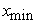and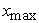are the minimum and maximum values among all values of the data. Additionally, the training can stop according to any one of the criteria of mean square error (MSE), the number of epochs of training or minimum performance gradient reaches certain value set as shown in Table 1. The MSE is calculated using Equation (6) below, where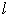is the total number of output neurons,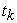is the target output and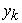is the network output. After the training is completed and new input data sets have to be evaluated, which have to be normalised too as the network has been trained in this way. Also, the output results from the network need to be denormalised using the inverse of the normalization function.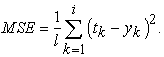(6)

#### Table 1. The used parameters for designing the neural networkDownload asPowerPoint Slide

Veiw figure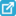View current table in a new window

The ANN learning process and the performance plot is depicted in Figure 11, which shows that the training with 487 epochs met the MSE stopping criteria (MSE less than 10E-4). Furthermore, it was established that the correlation coefficient (R) between the actual and desired (target) outputs has a value above 0.99 for the training, testing and validation data sets, which is indicative of a strong relationship between the outputs and targets of ANN. Thus, this network will be implemented for robot fault diagnosis.Download asVeiw figureFigures index
Figure 11. The ANN training process and performance plot

### 8. Performance Testing of the Designed SystemDownload asVeiw figureFigures index
Figure 12. Schematic diagram of the designed MLPN

With the ANN’s design satisfying the requirements and the training completed, its performance needs to be tested using sets of data not previously presented to the network, and however, the well-trained network must be able to show its capability in classifying the unseen data samples. The ANN has been trained in a way that produced results from the first neuron in the output layer of the ANN representing the healthy operating condition of the robot, while the remaining three neurons are mapped to represent the three simulated backlash faults on the robot, and thus a value of 1 for each output neuron is considered the target value. For instance, for the healthy case, the only output neuron that would have a value of 1 is the first one, therefore, it will be [1, 0, 0, 0]. The second neuron would take a value of 1 if the first fault type (high backlash) is presented and thus the outputs will be [0, 1, 0, 0], and so on with the other fault types, as illustrated in Figure 12. To test the network, a total of 80 unseen data samples were used. The first 20 samples represent the healthy condition of the robot while the remainder of the samples are divided into three groups of 20 samples each, corresponding to simulated robot faults. The test results are presented in Figure 13. The tests showed that the network efficiency in term of its capability in classifying the fpur different types of the robot health situation is 100 per cent, and the ANN design can differentiate the different faults of the robot with very good accuracy when confronted with unseen data.Download asVeiw figureFigures index
Figure 13. The designed ANN network performance testing with unseen healthy and faulty data

### 9. Conclusion

The development of the intelligent fault diagnosis system has been presented in this paper. From the review was established that two main categorizes of CM approaches are available: model-based and model-free approaches. In model-based CM, a model of the system (or of how it is presumed to behave) is created. Then, the predicted behaviour from this model is compared to the actual behaviour of the machine and any detected significant deviations can be interpreted as indications of faults. Accurate analytical models of industrial robots are often not practicable and difficult to be constructed due to dynamic complexity of the robot and unavoidable uncertainties. Accordingly, model-free approaches based on ANN has been considered for establishing the intelligent monitoring system of the robot. However, to design a reliable monitoring system, appropriate signal analysis techniques have to be employed, since these have a significant impact on the sensitivity of the features extracted from the signals captured. Wavelet analysis represents an efficient method for non-stationary signal analysis and therefore was used here.

In the experimental the PUMA 560 robot was used; three single axis, MEMS type accelerometers were utilized to measure the robot vibration in three axes (X, Y and Z). The optimum location that can be used to affix the accelerometers on the robot was justified and found to be close to the robot wrist. Joint three (elbow) of the robot was selected to simulate different backlash faults. The mechanical construction of this joint was comprehensively assessed to establish its assembly/disassembly, which has helped in understanding how the backlash level can be controlled. A discussion about different types of faults that may appear in the joint is also included, and the physical simulation and measurement of backlash were presented. Hybrid programming combining LabVIEW graphical programming with Matlab textural programming has been shown to be an effective method to build a signal monitoring and feature extraction system. To design an appropriate ANN and then evaluate its performance for the fault diagnosis, code was developed based on Matlab neural network toolbox. The ANN was trained to distinguish among different types of faults in the robot. A significant level of accuracy in fault diagnosis the ANN has been obtained and the percentage of correct classification was approximately 100%. The logical direction to further extend the capabilities of the designed system is to develop it to diagnosis other categories of faults that are related to joint motor, bearings and gears. The examined faults scenarios were simple, as only one fault is seeded at a time; however complex scenarios could be studied by combining multiple fault types at the same time. Also, in the present study, accelerated fault types were seeded in the joint gearbox. For future research, the algorithm could be extended to monitor the progressive development of faults in the robot transmission. This can be achieved by allowing the robot to operate for a considerable time period and the vibration level is monitored. Otherwise, a control device that can be connected to the backlash adjustment grub-screws and allow the progressive increase or decrease of the gear backlash while the robot is running could be developed.

### References

  M. W. Spong, S. Hutchinson, and M. Vidyasagar, Robot Modeling and Control: Wiley, 2005.In article  R. S. Beebe, Predictive Maintenance of Pumps Using Condition Monitoring. New York: Elsevier Advanced Technology, 2004.In article  V. F. Filaretov, M. K. Vukobratovic, and A. N. Zhirabok, “Observer-based fault diagnosis in manipulation robots,” Mechatronics, vol. 9, pp. 929-939, 1999.In article View Article  D. Brambilla, L. M. Capisani, A. Ferrara, and P. Pisu, “Actuators and sensors fault detection for robot manipulators via second order sliding mode observers,” 2008, pp. 61-66.In article  H. Liu, T. Wei, and X. Wang, “Signal decomposition and fault diagnosis of a scara robot based only on tip acceleration measurement,” 2009, pp. 4811-4816.In article  I. Trendafilova and H. Van Brussel, “Condition monitoring of robot joints using statistical and nonlinear dynamics tools,” Meccanica, vol. 38, pp. 283-295, 2003.In article View Article  A. Jaber and R. Bicker, “Industrial Robot Fault Detection Based on Wavelet Transform and LabVIEW,” in IEEE First International Conference on Systems Informatics, Modelling and Simulation, Sheffield, United Kingdom, 2014.In article  A. A. Jaber and R. Bicker, “The Optimum Selection of Wavelet Transform Parameters for the Purpose of Fault Detection in an Industrial Robot,” in 2014 IEEE - 4th International Conference on Control System, Computing and Engineering, Malaysia 2014.In article View Article  A. A. Jaber and R. Bicker, “Real-Time Wavelet Analysis of a Vibration Signal Based on Arduino-UNO and LabVIEW,” International Journal of Materials Science and Engineering, vol. 3, pp. 66-70, 2015.In article View Article  R. Bicker, A. Daadbin, and J. Rosinski, “The monitoring of vibration in industrial robots,” in ASME 12th Biennial Conference on Mechanical Vibration and Noise, 1989.In article  M. C. Pan, H. Van Brussel, and P. Sas, “Intelligent joint fault diagnosis of industrial robots,” Mechanical Systems and Signal Processing, vol. 12, pp. 571-588, 1998.In article View Article  J. T. Sawicki, A. K. Sen, and G. Litak, “Multiresolution wavelet analysis of the dynamics of a cracked rotor,” International Journal of Rotating Machinery, vol. 2009, 2009.In article  F. Al-Badour, M. Sunar, and L. Cheded, “Vibration analysis of rotating machinery using time-frequency analysis and wavelet techniques,” Mechanical Systems and Signal Processing, vol. 25, pp. 2083-2101, 2011.In article View Article  S. Debdas, M.F.Quereshi, A.Reddy, D.Chandrakar, and D.Pansari, “A Wavelet based multiresolution analysis for real time condition monitoring of AC machine using vibration analysis,” International Journal of Scientific and Engineering Research, vol. 2, 2011.In article  E. L. A. Vivas, A. Garcia-Gonzalez, I. Figueroa, and R. Q. Fuentes, “Discrete Wavelet transform and ANFIS classifier for Brain-Machine Interface based on EEG,” in 2013 6th International Conference on Human System Interactions, HSI 2013, 2013, pp. 137-144.In article View Article  A. Ghods and H. H. Lee, “A frequency-based approach to detect bearing faults in induction motors using discrete wavelet transform,” in Proceedings of the IEEE International Conference on Industrial Technology, 2014, pp. 121-125.In article View Article  M. Misiti, Y. Misiti, G. Oppenheim, and J.-M. Poggi, Wavelet Toolbox For Use with MATLAB: MathWorks, 1997.In article  W. L. Lim., “The application of artificial neural networks for sensor validation in diesel engine condition monitoring and fault diagnosis,” M. Phil., School of Civil Engineering and Geosciences, University of Newcastle upon Tyne, UK, 2009.In article  M. Misiti, Y. Misiti, G. Oppenheim, and J.-M. Poggi, Wavelet Toolbox for Use With Matlab: MathWorks, 2001.In article  A. Datta, C. Mavroidis, J. Krishnasamy, and M. Hosek, “Neural netowrk based fault diagnostics of industrial robots using wavelt multi-resolution analysis,” in American Control Conference, USA, 2007, pp. 1858-1863.In article View Article  A. R. Mohanty, MACHINERYCONDITION MONITORING: PRINCIPLES AND PRACTICES: Taylor & Francis Group, 2015.In article  J. Halme, “Condition monitoring of a material handling industrial robot,” in 19th Internation Congress, Lulea,Sweden, 2006.In article  British-Standard, “Gears — Cylindrical involute gears and gear pairs — Concepts and geometry,” ed, 2007.In article  C. Rodriguez-Donate, L. Morales-Velazquez, R. A. Osornio-Rios, G. Herrera-Ruiz, and R. J. Romero-Troncoso, “FPGA-based fused smart sensor for dynamic and vibration parameter extraction in industrial robot links,” Sensors, vol. 10, pp. 4114-4129, 2010.In article View Article  PubMed  M. Negnevitsky, Artificial intelligence: a guide to intelligent systems, Second ed.: ADDISON WESLEY, 2005.In article  J. Mazumdar, “SYSTEM AND METHOD FOR DETERMINING HARMONIC CONTRIBUTIONS FROM NONLINEAR LOADS IN POWER SYSTEMS,” Ph.D Thesis, Electrical and Computer Engineering, Georgia Institute of Technology, USA, 2006.In article  D. Pandya, S. UPADHYAY, and S. Harsha, “Ann based fault diagnosis of rolling element bearing using time-frequency domain feature,” International Journal of Engineering Science and Technology, vol. 4, pp. 2878-2886, 2012.In article  J. Heaton. (2008). The Number of Hidden Layers.In article  S.N.Sivanandam., S.Sumathi., and S.N.Deepa., Introduction to Neural Networks Using MATLAB 6.0: McGraw Hill, 2006.In article PubMed  P. Subbaraj and B. Kannapiran, “Fault detection and diagnosis of pneumatic valve using Adaptive Neuro-Fuzzy Inference System approach,” Applied Soft Computing Journal, vol. 19, pp. 362-371, 2014.In article View Article